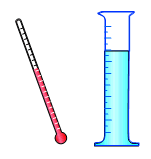Ch 4. The Mean Value Theorem Multimedia Engineering Math Maximum & Minimum Rolle'sTheorem Mean ValueTheorem MonotonicFunctions First DerivativeTest Concavity &Inflection SecondDerivative Test
 Chapter 1. Limits 2. Derivatives I 3. Derivatives II 4. Mean Value 5. Curve Sketching 6. Integrals 7. Inverse Functions 8. Integration Tech. 9. Integrate App. 10. Parametric Eqs. 11. Polar Coord. 12. Series Appendix Basic Math Units Search eBooks Dynamics Fluids Math Mechanics Statics Thermodynamics Author(s): Hengzhong Wen Chean Chin Ngo Meirong Huang Kurt Gramoll ©Kurt GramollMATHEMATICS - CASE STUDY IntroductionExperiment Equipments A group of college students in a chemistry lab are asked to find the maximum mass density of an unknown liquid between 0oC and 40oC. After they performed the experiment multiple times at different temperature, they determined the volume is a function of temperature. They summerized the formula to calculate the volume v of 1 kg at temperature t. It is     v = 999.87 - 0.06426T + 0.0085T2 - 0.00006T3 Questions At what temperature is the density a maximum? Approach The critical point of the mass density and the volume are the same. Maximum and minimum values may be located at critical points or boundary.